Courses

# Short Answer Type Questions (Part-1) - Arithmetic Progressions Class 10 Notes | EduRev

## Class 10 : Short Answer Type Questions (Part-1) - Arithmetic Progressions Class 10 Notes | EduRev

The document Short Answer Type Questions (Part-1) - Arithmetic Progressions Class 10 Notes | EduRev is a part of the Class 10 Course Class 10 Mathematics by VP Classes.
All you need of Class 10 at this link: Class 10

Note: For an A.P. with the 1st term and common difference ‘a’ and ‘d’ respectively, we have :
(a) nth term from the end = (m – n +1)th term from the beginning, where m is the number of terms in the A.P.
⇒ nth term from the end = (a) + (m – n)d
(b) If ‘l’ is the last term of the A.P., then
nth term from the end is the nth term of an A.P. whose first term is ‘l’ and the common difference is ‘–d’
⇒ nth term from the end = l + (n – 1) (–d)

Q1. If 9th term of an A.P. is zero, prove that its 29th term is double of its 19th term
Sol. Let ‘a’ be the first term and ‘d’ be the common difference.
Now, Using Tn = a + (n - 1) d, we have
T9 = a + 8d ⇒ a + 8d = 0 ...(1) [∵ T9 = 0 Given]
T19 = a + 18d = (a + 8d) + 10d = (0) + 10d = 10d  ...(2)
[∵ a + 8d = 0]
T29 = a + 28d
= (a + 8d) + 20d
= 0 + 20d = 20d   [∵ a + 8d = 0]
= 2 × (10d) = 2 (T19) [∵ T19 = 10d]
⇒ T29 = 2 (T19)
Thus, the 29th term of the A.P. is double of its 19th term.

Q2. If Tn = 3 + 4n then find the A.P. and hence find the sum of its first 15 terms.
Sol. Let the first term be ‘a’ and the common difference be ‘d’.
∵ Tn = a + (n - 1) d
∴ T1 = a + (1 - 1) d = a + 0 × d = a
T2 = a + (2 - 1) d = a + d
But it is given that
Tn = 3 + 4n
∴ T1 = 3 + 4 (1) = 7
⇒ First term, a = 7
Also, T2 = a + d = 3 + 4 (2) = 11
∴d = T2 - T1 = 11 - 7 = 4
Now, using Sn = n/2 [2a + (n - 1) d],
we get
S15 = 15/2 [2 (7) + (15 - 1) × 4]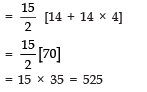Thus, the sum of the first 15 terms = 525.

Q3. Which term of the A.P.:
3, 15, 27, 39, ..... will be 120 more than its 53rd term?
Sol. The given A.P. is:
3, 15, 27, 39, .....
∴ a = 3
d = 15 - 3 = 12
∴ Using, Tn = a + (n - 1) d, we have:
T53 = 3 + (53 - 1) × 12
= 3 + (52 × 12)
= 3 + 624 = 627
Now, T53 + 120 = 627 + 120 = 747.
Let the required term be Tn
∴ Tn = 747
or a + (n - 1) d = 747
∴ 3 + (n - 1) × 12 = 747
⇒ (n - 1) × 12 = 747 - 3 = 744
⇒ n - 1 = 744/12 = 62
⇒ n = 62 + 1 = 63
Thus, the 63rd term of the given A.P. is 120 more than its 53rd term.

Q4. Find the 31st term of an A.P. whose 10th term is 31 and the 15th term is 66.
Sol. Let the first term is ‘a’ and the common difference is ‘d’.
Using Tn = a + (n - 1) d, we have:
T10 = a + 9d
⇒ 31 = a + 9d    ...(1)
Also T15 = a + 14d
⇒ 66 = a + 14d   ...(2)
Subtracting (1) from (2), we have:
a + 14d - a - 9d = 66 - 31
⇒ 5d = 35
⇒ d = 35/5 = 7
∴ From (1), a + 9d = 31
⇒ a + 9 (7) = 31
⇒ a + 63 = 31
⇒ a = 31 - 63
⇒ a = - 32
Now, T31 = a + 30d
= - 32 + 30 (7)
= - 32 + 210 = 178
Thus, the 31st term of the given A.P. is 178.

Q5. If the 8th term of an A.P. is 37 and the 15th term is 15 more than the 12th term, find the A.P. Hence find the sum of the first 15 terms of the A.P.
Sol. Let the 1st term = a
And the common difference = d
∴ Using Tn = a + (n - 1) d
∴ T8 = a + 7d
⇒ 37 = a + 7d    ...(1)
Also T15 = a + 14d
And T12 = a + 11d
According to the question,
T15 = T12 + 15
⇒ a + 14d = a + 11d + 15
⇒ a - a + 14d - 11d = 15
⇒ 3d = 15 ⇒ d = 15/3 = 5
From (1), we have:
a + 7 (5) = 37
⇒ a + 35 = 37
⇒ a = 37 - 35 = 2
Since an A.P. is given by :
a, a + d, a + 2d, a + 3d, ....
∴ The required A.P. is given by 2, 2 + 5, 2 + 2(5),... 2, 7, 12, ...
Now, using Sn = n/2 [2a + (n - 1) d]
∴ S15 = n/2 [2 (2) + 14 × 5]
= 15/2 [4 + 70]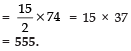Q6. The 5th and 15th terms of an A.P. are 13 and - 17 respectively. Find the sum of first 21 terms of the A.P.
Sol. Let ‘a’ be the first term and ‘d’ be the common difference.
∴ Using Tn = a + (n - 1) d, we have:
T15 = a + 14d = - 17    ...(1)
T5 = a + 4d = 13   ...(2)
Subtracting (2) from (1), we have:
(T15 - T5) = - 17 - 13 = - 30
⇒ a + 14d - a - 4d = - 30
⇒ 10d = - 30 ⇒ d = - 3
Substituting d = - 3 in (2), we get
a + 4d = 13
⇒ a + 4 (- 3) = 13
⇒ a + (- 12) = 13
⇒ a = 13 + 12 = 25
Now using Sn = n/2 [2a + (n - 1) d] we have:
S21 = 21/2 [2 (25) + (21 - 1) × (- 3)]
= 21/2[50 + (- 60)]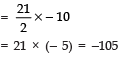Thus, the sum of the first fifteen terms = - 105.

Q7. The 1st and the last term of an A.P. are 17 and 350 respectively. If the common difference is 9 how many terms are there in the A.P.? What is their sum?
Sol. Here, first term, a = 17
Last term Tn = 350 = l
∵ Common difference (d) = 9.
∴ Using Tn = a + (n - 1) d, we have:
350 = 17 + (n - 1) × 9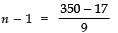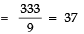⇒ n = 37 + 1 = 38

Thus, there are 38 terms.
Now, using, Sn = n/2 [a + l], we have
S38 = 38/2 [17 + 350]
= 19  = 6973
Thus, the required sum of 38 terms
= 6973.

Q8. If the sum of the first 7 terms of an A.P. is 49 and that of the first 17 terms is 289, find the sum of n terms.
Sol. Let the first term = a and the common difference = d.
∴ Using Sn = n/2 [2a + (n - 1) d]
∴ S7 = 7/2 [2a + 6d] = 49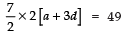⇒ 7 [a + 3d] = 49
⇒ a + 3d = 49/7 = 7
i.e., a + 3d = 7       ...(1)
Also S17 = 17/2 [2a + 16d] = 289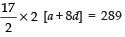⇒ 17 [a + 8d] = 289
⇒ a + 8d = 289/17 = 17
⇒ a + 8d = 17    ...(2)
Subtracting (2) from (1), we have:
a + 8d - a - 3d = 17 - 7
⇒ 5d = 10 ⇒ d = 2
From (1), we have
a + 3 (2) = 7
⇒ a + 6 = 7 ⇒ a = 7 - 6 = 1
Now, Sn = n/2 [2a + (n - 1) d]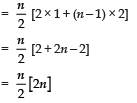Thus, the sum of n terms is n2.

Q9. The first and last term of an A.P. is 4 and 81 respectively. If the common difference is 7, how many terms are there in the A.P. and what is their sum?
Sol. Here, first term = 4 ⇒ a = 4 and d = 7.
Last term, l = 81 ⇒ Tn = 81
∵ Tn = a + (n - 1) d
∴ 81 = 4 + (n - 1) × 7
⇒ 81 - 4 = (n - 1) × 7
⇒ 77 = (n - 1) × 7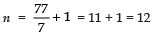⇒ There are 12 terms.
Now, using
Sn = n/2 (a + l)
⇒ S12 = 12/2 (4 + 81)
⇒ S12 = 6 × 85 = 510
∴ The sum of 12 terms of the A.P. is 510.

Q10. The angles of a quadrilateral are in A.P. whose common difference is 15°. Find the angles.
Sol. Let one of the angles = a
∵ The angles are in an A.P.
∴ The angles are:
a°, (a + d)°, (a + 2d)° and (a + 3d)°
∵ d = 15 [Given]
∴ The angles are:
a, (a + 15), [a + 2 (15)] and [a + 3 (15)]
i.e., a, (a + 15), (a + 30) and (a + 45).
∵ The sum of the angles of a quadrilateral is 360°.
∴ a + (a + 15) + (a + 30) + (a + 45) = 360°
⇒ 4a + 90° = 360°
⇒ 4a = 360° - 90° = 270°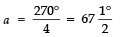∴ The four angles are: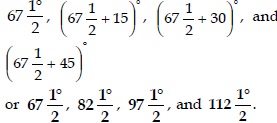Q11. The angles of a triangle are in AP. The greatest angle is twice the least. Find all the angles of the triangle.
Sol. Let a, b, c are the angles of the triangle, such that
c = 2a   ...(1)
Since a, b, c are in A.P.
Then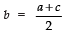...(2)
From (1) and (2), we get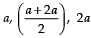are the three angles of the triangle.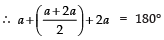⇒2a + a + 2a + 4a = 360°
⇒ 9a = 360°

⇒ a =  360°/9 = 40°

∴ The smallest angle  = 40°
The greatest angle = 2a = 2 × 40° = 80° The third angle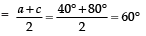Thus the angles of the triangle are 40°, 60°, 80°.

Q12. Find the middle term of the A.P. 10, 7, 4, ....., - 62.
Sol. Here, a = 10
d = 7 - 10 = - 3
Tn = (- 62)
∴ Using Tn = a + (n - 1) d, we have
- 62 = 10 + (n - 1) × (- 3)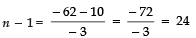⇒ n = 24 + 1 = 25
⇒ Number of terms = 25
∴ Middle term =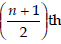term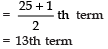Now T13 = 10 + 12d
= 10 + 12 (- 3)
= 10 - 36 = - 26
Thus, the middle term = - 26.

Q13. Find the sum of all three digit numbers which are divisible by 7.
Sol. The three digit numbers which are divisible by 7 are:
105, 112, 119, ....., 994.
It is an A.P. such that
a = 105
d = 112 - 105 = 7
Tn = 994 = l
∵ Tn = a + (n - 1) × d
∴ 994 = 105 + (n - 1) × 7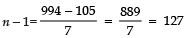⇒ n = 127 + 1 = 128
Now, using Sn = n/2 [a + l]
We have S128 = 128/2[105 + 994]
= 64 
= 70336
Thus, the required sum = 70336.

Q14. Find the sum of all the three digit numbers which are divisible by 9.
Sol. All the three digit numbers divisible by 9 are:
117, 126, ....., 999 and they form an A.P.
Here, a = 108
d = 117 - 108 = 9
Tn = 999 = l
Now, using Tn = a + (n - 1) d, we have
999 = 108 + (n - 1) (9)
⇒ 999 - 108 = (n - 1) × 9
⇒ 891 = (n - 1) × 9
⇒ n - 1 = 891/9 = 99
⇒ n = 99 + 1 = 100
Now, the sum of n term of an A.P. is given
Sn = n/2 [a + l]
∴ S100 = 100/2[108 + 999]
= 50 
= 55350
Thus, the required sum is 55350.

Q15. Find the sum of all the three digit numbers which are divisible by 11.
Sol. All the three digit numbers divisible by 11 are 110, 121, 132, ....., 990.
Here, a = 110
d = 121 - 110 = 11
Tn = 990
∴ Using Tn = a + (n - 1) d, we have
990 = 110 + (n - 1) × 11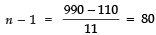⇒ n = 80 + 1 = 81

Now, using Sn = n/2 [a + l], we have
S81 = 81/2 [110 + 990]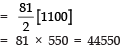Thus, the required sum = 44550.

Q16. The sum of the first six terms of an AP is 42. The ratio of the 10th term to its 30th term is 1:3. Calculate the first term and 13th term of A.P.
Sol. ∵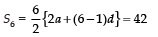∴ 6a + 15d = 4 2    ...(1)
Also, (a10) : (a30) = 1 : 3
or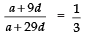⇒ 3(a + 9d) = a + 29d
⇒ 3a + 27d = a + 27d
⇒ 2a = 2 d

⇒ a = d     ...(2)
From (1) 6d + 15d = 42 ⇒ d = 2
From (2) a = d ⇒ d = 2
Now, a13 = a + 12d
= 2 + 12 × 2 = 26

Offer running on EduRev: Apply code STAYHOME200 to get INR 200 off on our premium plan EduRev Infinity!

132 docs

,

,

,

,

,

,

,

,

,

,

,

,

,

,

,

,

,

,

,

,

,

;